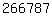## Robert M. Strain# Robert M. Strain

##### PhD, Brown University, Division of Applied Mathematics, 2005

Welcome to my homepage. I am a professor in the Department of Mathematics, and an affiliated faculty member of the Applied Mathematics and Computational Science graduate program, at the University of Pennsylvania.

My research focuses on the mathematical analysis of non-linear partial differential equations which arise in physical contexts. I have proven results on partial differential equations from diverse areas including fluid dynamics, kinetic theory, and materials science. I do research on problems involving local and global existence and uniqueness of solutions, large time sharp asymptotic behavior and convergence to equilibrium, finite time blow up, and ill-posedness of solutions. I tend to study physically motivated partial differential equations including the incompressible Navier-Stokes equations, the relativistic Euler system, the Muskat problem, the Boltzmann and Landau equation under Newtonian mechanics or special-relativity and the Vlasov equations.

I currently serve on the Editorial Boards of the following journals: Acta Applicandae Mathematicae (ACAP), Communications in Mathematical Analysis and Applications (CMAA), Communications on Pure and Applied Analysis (CPAA), and SIAM Journal on Mathematical Analysis (SIMA).

### Contact Information

Department of Mathematics
University of Pennsylvania
David Rittenhouse Lab
209 South 33rd Street